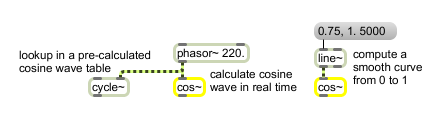# cos~

Signal cosine function (0-1 range)

## Description

cos~ will take any given signal and output a signal which is the cosine function of each sample within the input signal.

None.

## Messages

 signal Input to a cosine function. The input is stated as a fraction of a cycle (typically in the range from 0 to 1), and is multiplied by 2π before being used in the cosine function.

## Output

signal: The cosine of 2π times the input. The method used in this object to calculate the cosine directly is typically less efficient than using the stored cosine in a cycle~ object.

## ExamplesCosine of the input (a fraction of a cycle) is calculated and sent out

Name Description
acos~ Signal arc-cosine function
acosh~ Signal hyperbolic arc-cosine function
asin~ Signal arc-sine function
asinh~ Signal hyperbolic arc-sine function
atan~ Signal arc-tangent function
atanh~ Signal hyperbolic arc-tangent function
atan2~ Signal arc-tangent function (two variables)
cosh~ Signal hyperbolic cosine function
cosx~ Signal cosine function
cycle~ Table lookup oscillator
phasor~ Sawtooth wave generator
sinh~ Signal hyperbolic sine function
sinx~ Signal sine function
tanh~ Signal hyperbolic tangent function
tanx~ Signal tangent function
trapezoid~ Trapezoidal wavetable
triangle~ Triangle/ramp wavetable
wave~ Variable size wavetable
2d.wave~ Two-dimensional wavetable